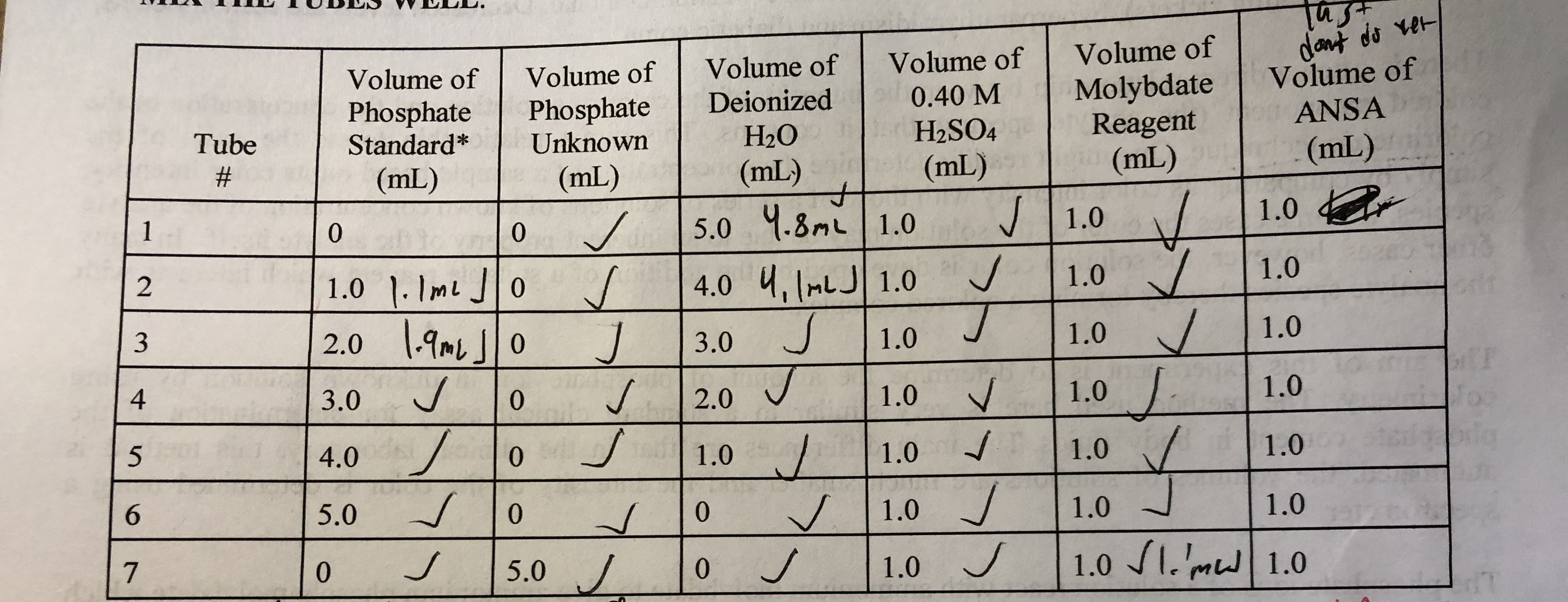Volume of Volume of Volume of Volume of Volume ofPhosphate | Phosphate | Deionized | 0.40M | Molybdate | Volume ofH2SO4Reagent ANSATubeStandardUnknown(mL)H2O(mL)(mL)1.01.01.0(mL)(mL)1.05.0 Ч.3mLI 1.04.0 1.0 1.001.0 .Jo1.043.04.05.002.01.01.01.001.01.01.0o 5.0/

Question

how would I calculate the molarity ( in units of micromolar) of the phosphate of tube 7, with the molarity of phosphate being 250 micromoles/liter?help_outlineImage TranscriptioncloseVolume of Volume of Volume of Volume of Volume of Phosphate | Phosphate | Deionized | 0.40M | Molybdate | Volume of H2SO4Reagent ANSA TubeStandardUnknown (mL) H2O (mL) (mL) 1.0 1.0 1.0 (mL) (mL) 1.0 5.0 Ч.3mLI 1.0 4.0 1.0 1.0 0 1.0 .Jo 1.0 4 3.0 4.0 5.0 0 2.0 1.0 1.0 1.0 0 1.0 1.0 1.0 o 5.0/ fullscreen
Step 1

Number of moles of a substance refer to the unit of amount. One mole of a substance can be defined as the mass or quantity of given substance which contains same number of fundamental units as there are number of atoms in 12.000g of 12C. These fundamental units can be molecules, atoms or the formula units. It is denoted by symbol ‘n’. The formula to calculate number of moles is given as

Step 2

Molarity of a solution is used to describe the relationship between number of moles and volume of a given solution. It is denoted by symbol ‘M’ and the units of molarity are moles/liter or simply ‘M’. The formula for molarity is given as

Step 3

In the given question we have been given the molarity of phosphate  solution to be 250.0 micromo...

Want to see the full answer?

See Solution

Want to see this answer and more?

Our solutions are written by experts, many with advanced degrees, and available 24/7

See Solution
Tagged in

Chemistry